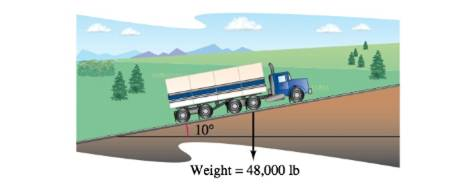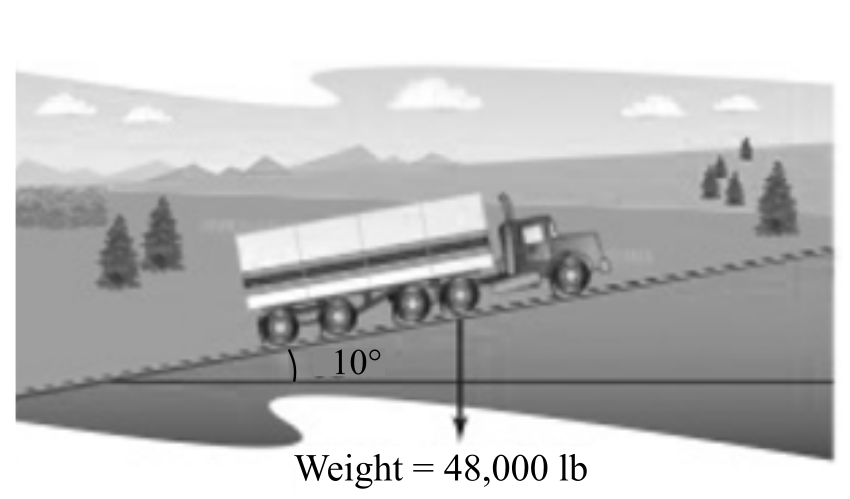Chapter 11.3, Problem 59E

Chapter
Section
Textbook Problem

# Braking Load A 48,000-pound truck is parked on a 10° slope (see figure). Assume the only force to overcome is that due to gravity. Find (a) the force required to keep the truck from rolling down the hill and (b) the force perpendicular to the hill.(a)

To determine

To calculate: The amount of the force required to keep the 48, 000-pound truck on a 10° slope from rolling down the hill.Explanation

Given:

A 48,000-pound truck is parked on a 10° slope and the only force to overcome is that due to gravity.

Formula used:

The work W done by a constant force F as its point of application moves along the vector v is given by W=(Fvv2)v

Calculation:

Gravitational force vector; F=48000j.

The unit vector to the truck position can be written as v=cos(10°)i+sin(10°)j.

So,

v=cos210°+sin210°=1

To find the force to keep it from rolling down the hill, now to find the gravitational force component along the slope, or component of F along v

(b)

To determine

To calculate: The force perpendicular to the hill when a 48,000-pound truck is parked on a 10° slope.### Still sussing out bartleby?

Check out a sample textbook solution.

See a sample solution

#### The Solution to Your Study Problems

Bartleby provides explanations to thousands of textbook problems written by our experts, many with advanced degrees!

Get Started

#### Find more solutions based on key concepts